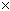SEARCH HOMEMath Central Quandaries & QueriesQuestion from MITCH, a parent: CAN YOU GIVE ME A LIST OF ALL POSSIBLE FOUR DIGIT COMBINATIONS USING 0 TO 9 NO REPEAT NUMBERS AND THE NUMBERS DO NOT HAVE TO BE ENTERED IN A SPECIAL ORDER IF YOU COULD ALSO SHOW ME HOW TO CALULATE THIS IT WOULD BE GREAT THANKS MITCHHi Mitch.

I cannot give you a list, but I can tell you how it is calculated.

1. Pick any number from 0-9 to go into the first (thousands) spot. There are ten digits, so you have ten choices.
2. Since you cannot re-use that number, there are nine possible digits left from which you pick one to go in the second (hundreds) spot.
3. Likewise you have eight choices for the third (tens) spot
4. And seven choices for the fourth (units) spot.

Now you have to apply the Fundamental Counting Principle:

If there are m ways to do one thing, and n ways to do another, then there are mn ways of doing both.

What this means for us is that if there are 10 ways of choosing the first digit and 9 ways of choosing the second digit, then there are 109 ways of choosing the first two digits. It continues: if there are 109 ways of choosing the first pair of digits and 8 ways of choosing the third digit, there are 1098 ways of choosing the first three digits. And so on...

5. So now you multiply the choices together: 10987 to get the number of different four digit sequences where order does matter.

To make it unordered, we have find the number or ways a given set of four digits can be re-arranged. We use the Fundamental Counting Principle again. The first digit will be one of the four we chose. The second digit will be one of the three that are left, and so on. So there are 4321 ways of ordering a four-digit number.

6. Finally, we divide the number of "ordered" possibilities by the duplication factor (the number of ways any one group of four digits can be re-arranged). So your answer is (10987) / (4321).

Hope this helps,
Stephen La Rocque.Math Central is supported by the University of Regina and The Pacific Institute for the Mathematical Sciences.